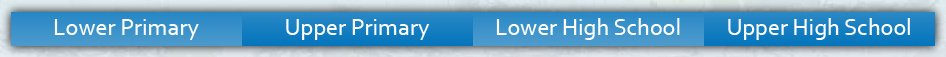Upper Primary Enter keywords to search by topic or worksheet description

Worksheets in this category help students to read, write and understand common fractions and mixed numbers, and to be able to apply the four operations to solve problems involving fractions.

Worksheet Name Files
Fractions: Writing in Words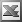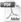Fractions: Equivalent Fractions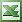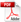Fractions: Comparing Using >, <, =Fractions: Arranging in OrderAdding Fractions: Like DenominatorsAdding Fractions: Related DenominatorsAdding Fractions: Mixed DenominatorsSubtracting Fractions: Like DenominatorsSubtracting Fractions: Related DenominatorsSubtracting Fractions: Mixed DenominatorsMultiplying FractionsDividing FractionsFraction Review: Four Operations on FractionsConverting Mixed Numbers to Improper FractionsConverting Improper Fractions to Mixed NumbersFraction of a QuantityFractions: Naming Fractions from Diagrams 1Fractions: Naming Fractions from Diagrams 2